# Excel trendline types, equations and formulas

In this tutorial, you will find the detailed description of all the trendline options available in Excel and when to use them. You will also learn how to display a trendline equation in a chart and find the slope of trendline.

It is very easy to add a trendline in Excel. The only real challenge is to choose the trendline type that best corresponds to the type of data you are analyzing. In this tutorial, you will find the detailed description of all the trendline options available in Excel and when to use them. If you are looking for how to insert a trendline in an Excel chart, please check out the above linked tutorial.

## Excel trendline types

When adding a trendline in Excel, you have 6 different options to choose from. Additionally, Microsoft Excel allows displaying a trendline equation and R-squared value in a chart:

• Trendline equation is a formula that finds a line that best fits the data points.
• R-squared value measures the trendline reliability - the nearer R2 is to 1, the better the trendline fits the data.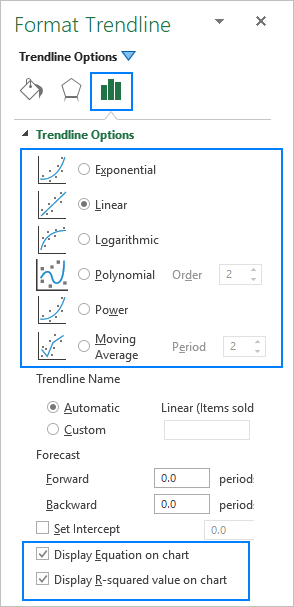Below, you will find a brief description of each trendline type with chart examples.

### Linear trendline

The linear trend line is best to be used with linear data sets when the data points in a chart resemble a straight line. Typically, a linear trendline describes a continuous rise or fall over time.

For example, the following linear trendline shows a steady increase in sales over 6 months. And the R2 value of 0.9855 indicates a pretty good fit of the estimated trendline values to the actual data.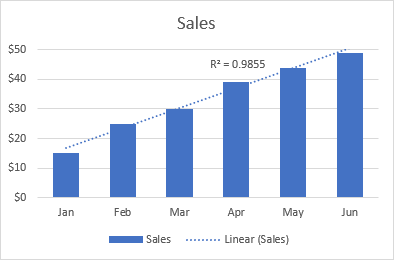### Exponential trendline

The exponential trendline is a curved line that illustrates a rise or fall in data values at an increasing rate, therefore the line is usually more curved at one side. This trendline type is often used in sciences, for example to visualize a human population growth or decline in wildlife populations.

Please note that an exponential trendline cannot be created for data that contains zeros or negative values.

A good example of an exponential curve is the decay in the entire wild tiger population on the earth.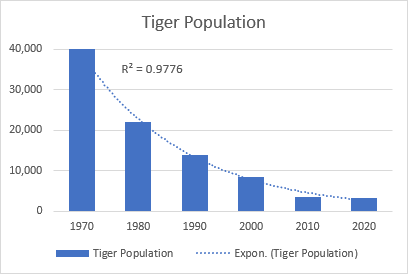### Logarithmic trendline

The logarithmic best-fit line is generally used to plot data that quickly increases or decreases and then levels off. It can include both positive and negative values.

An example of a logarithmic trendline may be an inflation rate, which first is getting higher but after a while stabilizes.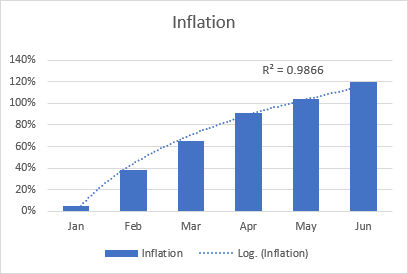### Polynomial trendline

The polynomial curvilinear trendline works well for large data sets with oscillating values that have more than one rise and fall.

Generally, a polynomial is classified by the degree of the largest exponent. The degree of the polynomial trendline can also be determined by the number of bends on a graph. Typically, a quadratic polynomial trendline has one bend (hill or valley), a cubic polynomial has 1 or 2 bends, and a quartic polynomial has up to 3 bends.

When adding a polynomial trendline in an Excel chart, you specify the degree by typing the corresponding number in the Order box on the Format Trendline pane, which is 2 by default: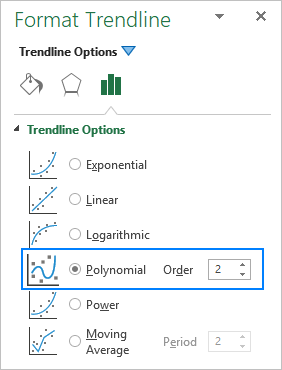For example, the quadratic polynomial trend is evident on the following graph that shows the relationship between the profit and the number of years the product has been on the market: rise in the beginning, peak in the middle and fall near the end.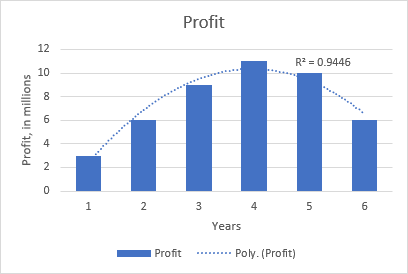### Power trendline

The power trend line is very similar to the exponential curve, only it has a more symmetrical arc. It is commonly used to plot measurements that increase at a certain rate.

A power trendline cannot be added to an Excel chart that contains zero or negative values.

As an example, let's draw a power trendline to visualize the chemical reaction rate. Note the R-squared value of 0.9918, which means that our trendline fits the data almost perfectly.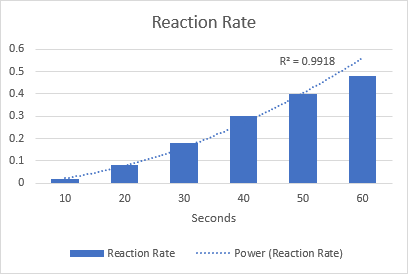### Moving average trendline

When the data points in your chart have a lot of ups and downs, a moving average trendline can smooth the extreme fluctuations in data values to show a pattern more clearly. For this, Excel calculates the moving average of the number of periods that you specify (2 by default) and puts those average values as points in the line. The higher the Period value, the smoother the line.

A good practical example is using the moving average trendline to reveal fluctuations in a stock price that otherwise would be difficult to observe.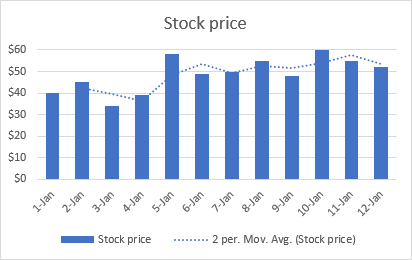## Excel trendline equations and formulas

This section describes the equations that Excel uses for different trendline types. You do not have to build these formulas manually, simply tell Excel to display the trendline equation in a chart.

Also, we will discuss the formula to find the slope of a trendline and other coefficients. The formulas assume that you have 2 sets of variables: independent variable x and dependent variable y. In your worksheets, you can use these formulas to get the predicted y values for any given values of x.

For consistency, we will be using the same data set with slightly varying values for all the examples. However, please keep in mind that it's only for demonstration purposes. In your real worksheets, you should choose the trendline type corresponding to your data type.

Important note! The trendline formulas should only be used with XY scatter charts because only this chart plots both x and y axes as numeric values. For more information, please see Why Excel trendline equation may be wrong.

### Linear trendline equation and formulas

The linear trendline equation uses the least squares methods to seek the slope and intercept coefficients such that:

y = bx + a

Where:

• b is the slope of a trendline.
• a is the y-intercept, which is the expected mean value of y when all x variables are equal to 0. On a chart, it's the point where the trendline crosses the y axis.

For linear regression, Microsoft Excel provides special functions to get the slope and intercept coefficients.

Slope of trendline
b: `=SLOPE(y,x)`

Y-intercept
a: `=INTERCEPT(y,x)`

Assuming the x range is B2:B13 and the y range is C2:C13, the real-life formulas go as follows:

`=SLOPE(C2:C13, B2:B13)`

`=INTERCEPT(C2:C13,B2:B13)`

The same results can be achieved by using the LINEST function as an array formula. For this, select 2 adjacent cells in the same row, enter the formula and press Ctrl + Shift + Enter to complete it:

`=LINEST(C2:C13,B2:B13)`

As shown in the screenshot below, the slope and intercept coefficients returned by the formulas are perfectly in line with the coefficients in the linear trendline equation displayed in the chart, only the latter are rounded to 4 decimal places: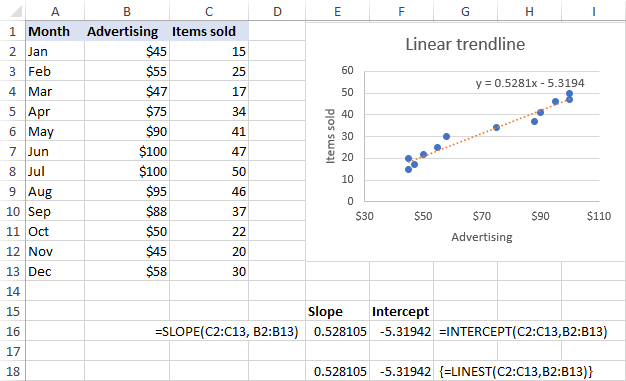### Exponential trendline equation and formulas

For the exponential trendline, Excel uses the following equation:

y = aebx

Where a and b are calculated coefficients and e is the mathematical constant e (the base of the natural logarithm).

The coefficients can be calculated by using these generic formulas:

a: `=EXP(INDEX(LINEST(LN(y), x), 1, 2))`

b: `=INDEX(LINEST(LN(y), x), 1)`

For our sample data set, the formulas take the following shape:

a: `=EXP(INDEX(LINEST(LN(C2:C13), B2:B13), 1, 2))`

b: `=INDEX(LINEST(LN(C2:C13), B2:B13), 1)`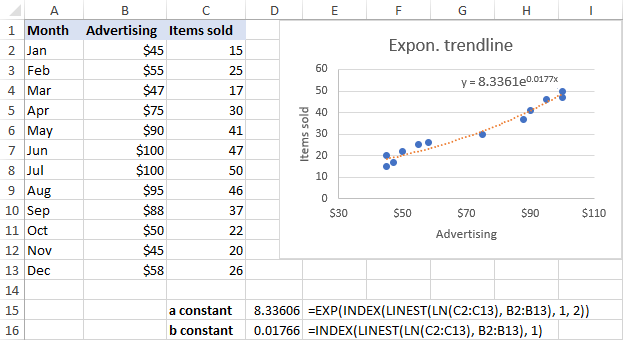### Logarithmic trendline equation and formulas

Here's the logarithmic trendline equation in Excel:

y = a*ln(x)+b

Where a and b are constants and ln is the natural logarithm function.

To get the constants, use these generic formulas, which only differ in the last argument:

a: `=INDEX(LINEST(y, LN(x)), 1)`

b: `=INDEX(LINEST(y, LN(x)), 1, 2)`

For our sample data set, we use these ones:

a: `=INDEX(LINEST(C2:C13, LN(B2:B13)), 1)`

b: `=INDEX(LINEST(C2:C13, LN(B2:B13)), 1, 2)`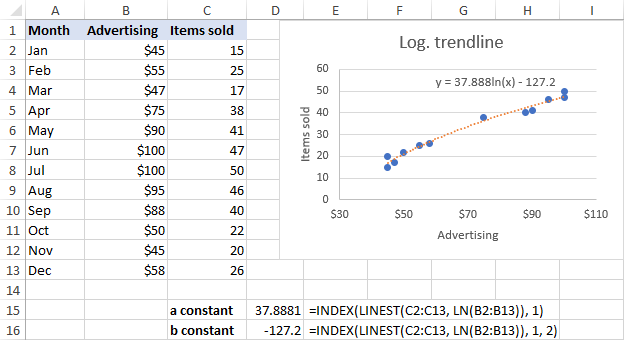### Polynomial trendline equation and formulas

To work out the polynomial trendline, Excel uses this equation:

y = b6x6 + … + b2x2 + b1x + a

Where b1b6 and a are constants.

Depending on the degree of your polynomial trendline, use one of the following sets of formulas to get the constants.

#### Quadratic (2nd order) polynomial trendline

Equation: y = b2x2+ b1x + a

b2: `=INDEX(LINEST(y, x^{1,2}), 1)`

b1: `=INDEX(LINEST(y, x^{1,2}), 1, 2)`

a: `=INDEX(LINEST(y, x^{1,2}), 1, 3)`

#### Cubic (3rd order) polynomial trendline

Equation: y = b3x3 + b2x2+ b1x + a

b3: `=INDEX(LINEST(y, x^{1,2,3}), 1)`

b2: `=INDEX(LINEST(y, x^{1,2,3}), 1, 2)`

b1: `=INDEX(LINEST(y, x^{1,2,3}), 1, 3)`

a: `=INDEX(LINEST(y, x^{1,2,3}), 1, 4)`

The formulas for higher degree polynomial trendlines can be built by using the same pattern.

For our data set, the 2nd order polynomial trendline suites better, so we are using these formulas:

b2: `=INDEX(LINEST(C2:C13, B2:B13^{1,2}), 1)`

b1: `=INDEX(LINEST(C2:C13, B2:B13^{1,2}), 1, 2)`

a: `=INDEX(LINEST(C2:C13, B2:B13^{1,2}), 1, 3)`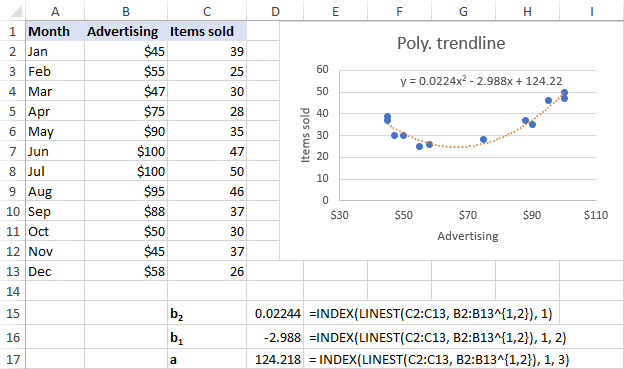### Power trendline equation and formulas

A power trendline in Excel is drawn based on this simple equation:

y = axb

Where a and b are constants, which can be calculated with these formulas:

a: `=EXP(INDEX(LINEST(LN(y), LN(x),,), 1, 2))`

b: `=INDEX(LINEST(LN(y), LN(x),,), 1)`

In our case, the following formulas work a treat:

a: `=EXP(INDEX(LINEST(LN(C2:C13), LN(B2:B13),,), 1, 2))`

b: `=INDEX(LINEST(LN(C2:C13), LN(B2:B13),,), 1)`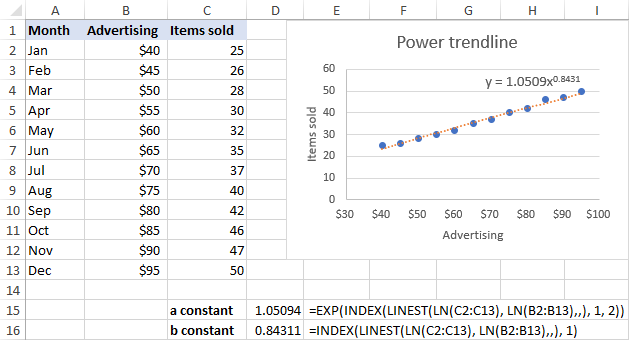## Excel trendline equation is wrong - reasons and fixes

If you think that Excel has drawn a trendline incorrectly or the trendline formula displayed in your chart is wrong, the following two points may shed some light on the situation.

### Excel trendline equation is correct only in scatter charts

Excel trendline formulas should only be used with XY (scatter) graphs because only in this chart type both the y-axis and x-axis are plotted as numeric values.

In line charts, column and bar graphs, numeric values are plotted only on the y-axis. The x-axis is represented by a linear series (1, 2, 3,…) regardless of whether the axis labels are numbers or text. When you make a trendline in these charts, Excel uses those assumed x-values in the trendline formula.

### Numbers are rounded in Excel trendline equation

To occupy less space in the chart, Excel displays very few significant digits in a trendline equation. Nice in terms of design, it significantly reduces the formula's accuracy when you manually supply x values in the equation.

An easy fix is to show more decimal places in the equation. Alternatively, you can calculate the coefficients by using a formula corresponding to your trendline type, and format the formula cells so that they show a sufficient number of decimal places. For this, simply click the Increase Decimal button on the Home tab in the Number group.

That's how you can make different trendline types in Excel and get their equations. I thank you for reading and hope to see you on our blog next week!

## You may also be interested in

### 59 comments to "Excel trendline types, equations and formulas"

1.Nhat says:

The Formula to extract the constants of Polynominal trendline only work in Vertical data table.
It not work in my Horizontal data table.
How to keep it working in Horizontal data table?

2.Armadillo says:

What if we want to find the coefficients to the trendline equation with the y-intercept set equal to a particular value (e.g., y-int. = 0)?

3.Tom Bjorklund says:

My trendline plots correctly but the trendline equation solution for y yields incorrect y values. The equation is a 6th-degree polynomial. The correct y values range from -20 to 80. The x values range from 1900 to 2050. The incorrect y values are all in the range of -2.38E+15, plus and minus. I increased the decimal value to 30, but that did not affect the outcome. Any suggestion on how to fix this problem?

4.Enzo says:

Thanks!
it works fine to me!
but only if the known_x and known_y vectors are columns... if they are rows instead, it gives me #VALUE error... is this normal/is there any way to use the function working with row input series?
Thanks!

5.Bill says:

Masterful job. College professors take note. Teach in understandable ways so that students can take practical knowledge with them. You don.t need to prove how smart you are.

6.Steve says:

I suspect that many of the #VALUE! errors (mine included) are the result of missing values, but I don't have time to investigate further. The cubic trendline function seems to not be troubled by missing values, but the coefficient extraction functions (INDEX and LINEST) generate #VALUE! errors on the same data series. Does anyone know how they treat missing values?

7.tom stakem says:

From a series of absolute numbers, which happen to be future quarterly estimates, I would like to calculate a growth rate. Is that possible and if so what would be the Excel formula code? merci

The numbers are: 2.91, 3.10, 3.28, 3.28, 3.52, 3.48, 3.38, 3.64, 4.12, 4.16, 3.72, 3.90, 3.68.

And if any of the numbers had negative values would that negate the exercise or is there a work around?

8.Bob Carr says:

This is very helpful information. I am confused though on how to calculate a value from the Power Law trendline in Excel. For instance, the trendline that I am looking at has values from 100 - 700 on the x-axis, 10,000 - 50,000 on the y-axis, and a curve that is asymptotic to both axes, decreasing from right to left. The provided trendline equation is y = 3E + 08x^(-1.611). What in the world does this mean?! Plugging any value of x into the equation produces a value of y = 3E + 0!

•JG says:

In case you still need an answer, y = 3E + 08x^(-1.611) means y = (3*10^8) * x^(-1.611).

9.Karl says:

Hi, thanks for this very useful guide. I have calculated the a,b,c coefficients for my binomial equation (y = ax2+bx+c). From here, how do I compare this model with my actual data and calculate the R2 ?

10.bob says:

problem solved - used log (x) as new column and then do straight polynomial fitting on that!

11.bob says:

Help... I am trying to extract all the coefficients of this formula: y=10^(ax^4+bx^3+cx^2+dx+e)

but not working (esp the array part of the input), could someone shed some light please?

thank you!

12.Zel says:

Thank-you this was exactly what I needed

13.Tom says:

glad to see people finding it useful, wish I could apply it too. I have a column of sales we've made thus far and have it plotted on a chart, where I also use plynomial trendline. how can I using the formulas above get the value of trendline at the end of period?

thanks, appriciate support

14.DAS says:

WHAT IS THE DIFFERENCE BETWEEN POLYNOMIAL ORDER 3 AND 4

•JG says:

Order 3 is y=Ax^3 + Bx^2 + Cx^1 + D. Order 4 is Order 3 is y=Zx^4 + Ax^3 + Bx^2 + Cx^1 + D.

15.Eloïse BONJEAN says:

great, thanks I tried with poly of order 3 (ax3 + bx2 + cx1 + x0)
it works perfectly and avoids me to make a trendline and manually copy the results
a possible improvement would be to allow the use of =na() for some empty cells (because I have differenet lines and x not always the same -results of measurements-) this is possible with graph

16.Stylianos Grigoriadis says:

Hello!

Thanks for the information they were extremely helpful although I have some issues:
1) In my excel I cannot use comma (,) but only (;) for codes, otherwise excel will not understand it is a code and not just writing,
2) When I change it from "," to ";" and run the equations for the a, b1, and b2 for the polyonomial trendline, if I have negative values I take the message #VALUE, while when I only have positive values I get a normal result.

How should I address those issues?

Thank you again for the help!

17.Jan Królikowski says:

=LINEST({2;6;10},{1;2;3})
work fine and calculate a,b for y=ax+b

=TREND({2;6;10},{1;2;3},4)
work fine and calculate next y

=LINEST({2;6;14},{1;2;3}^{1,2})
work fine and calculate a,b,c for y=ax^2+bx+c

=TREND({2;6;14},{1;2;3}^{1,2},4)
does not work! do you know why?

is there another TREND_FOR_POLYNOMINAL_OF_SECOND_ORDER function?

•Luda says:

Try following formula:
=TREND({2;6;14},{1;2;3}^{1,2},4^{1;2})

18.mike s says:

Thank you! This was a big help

19.Mijo says:

Hi Svetlana,
May I ask what does ^{1,2} and ^{1,2,3} stands for in Polynomial trendline formulas b2: =INDEX(LINEST(y, x^{1,2}), 1) and b3: =INDEX(LINEST(y, x^{1,2,3}), 1) ?

•Svetlana Cheusheva says:

Hi Mijo,

In Excel, the caret (^) is used for exponentiation, i.e. to raise a number to power. In our case, x^{1,2} raises each x value to the power of 1 and to the power of 2.

20.Hamdi says:

Hello

I am using the power trend line, and the equation is like this

y=a x^b ,

i want to fix b to have a value of 0.5
and the only changing is a.

any way how to do this.

21.Arsha says:

How to get equation for trigonometric (sinousoidal) graph in excel or Origin

22.Simon Christen says:

About exponential trendline it appears =RSQ(Ln(Known_y's),(Known_x's)) to calculate R^2

23.Nadeen says:

24.Chris says:

Fantastic help - Thank you

25.SHAD says:

Hi,

I have an equation must be solved by excel but im not aure what function to use. The question give me the following 4+y/(2x+y)^2+12. The given X=4 and y=6. And they said the X value and Y value cells i have to rename to be cell of 4 name box (x) and 6 name box (y) . What function i have to use to enter to be solved. Hi t the basic calculation method not accepted as manual. I tried normative and others but i cant reach a solution.

26.max says:

spasibo!

27.Vincent says:

Hi Svetlana,
Thank you for your sharing. I have applied the formula to find the coefficients of a polynomial trendline with degree 6 but the answer turns out to be wrong. Can you help me to solve this?

28.Vincent says:

Hi Svetlana,
I would like to ask if I want to find the coefficients of a polynomial trendline with degree 6, how should I do that? I applied the formula above and the answer was worry. Can u help me?

29.Pritt.Sweety says:

No matter how many times you fail, you must face life and be full of hope

30.Maurice LeBlanc says:

This is very useful, thank you!
I did get the formulas to work; however, I have a challenge with empty cells. I'm pulling data from a pivot table for a number interconnected y-values (ie. the sum of the y's should sum to a total) but there are voids throughout. If the array contains a void, the formula doesn't work.
Is there an easy way around this?

31.Kopal Tandon says:

Hi,

How can I make x=y in scatter plots in excel? I have two data series.

32.Anne says:

Thank you , this is clear, please share with us how to get the second part.

33.Anne says:

Thank you , this is clear, where is the second part.

34.lelia says:

what can i use if my data string includes values of 0?

35.Somchai says:

I have problem with trendline equation. The X value in the equation is not the same scale as the input values. My input X values are 2000, 3000, 4000, ..., but Excel use number 1,2,3, ... as the input value. How to correct the problem.

36.Bo Håkansson says:

How do I retrieve the real values of a Trendline in the easiest way for example peak values etc?

Bo

37.Johnny Ancich says:

This was a good explanation, but what I find impossible to find is how to calculate R2 for some of these curves (like logarithmic and power)

38.Henry Nguyen says:

Hi Svetlana,
I tried to lug the equation back to re-test the equation, but the result isn't the same. Can you help to explain why and how to fix it? Thanks.

•Svetlana Cheusheva says:

Hi Henry,

It's difficult to make any assumptions without seeing your data. The only thing that comes to mind is that Excel displays only a few significant digits in a trendline equation for the sake of space. You can try to display more digits as explained in How to show more decimal places in a trendline equation.

Also, please keep in mind that an trendline equation is correct only in scatter charts because only this chart type plots both the y-axis and x-axis as numeric values.

•Migo Bekhit says:

I tested all the formulas and compared them against the graph results and it's all working perfect. There are some variances as the graph formulas are rounded up which means those formulas are more accurate than the graph results.

Svetlana,
Thanks a lot for those amazing tips. It saved me a lot of time building charts.

39.Luis Amador says:

Is it possible to use an IF condition to control the display of a trendline on a scatterplot?, for example something along the lines of:
" =IF(A1==1,Show exponential trendline, IF(A1==2,show logarithmic trenline, show linear trendline)) "

40.Sergey says:

Works great, Thanks Светлана!
Is there any way to get R2 in a sheet cells, without building a chart and trendlines? E.g. you are saying polinominal 2 is more accurate than polinominal 3, but without comparing R2 you can not tell this. I need to show my boss that we have compared all different trendlines for this particular data and the range of R2 is as follow.. It is ok also if the answer will be no it can not be done...
This is very helpful website - thanks a lot for all you are doing.
Sergey.

•Svetlana Cheusheva says:

Hi Sergey,

The good news is that Excel has a built-in function to find R-Squared:
RSQ(known_y's,known_x's)

And thank you for your kind words!

•Sergey says:

Svetlana,
Thanks for your quick reply, the RSQ formula returns the square of the Pearson product moment correlation, but Pearson equation is only for linear correlations. SO I will not be able to use this equation to get R2 for the polinominal or any other trendlines except of the linear and compare the R2 for different trendlines.

Thanks,

•Svetlana Cheusheva says:

Sergey,
You are absolutely right. Sorry for my hasty response, I overlooked that you were looking to compare R2 of all different trendlines. In this case, I do not know a way to get R2 other than by building a chart.

•Spencer Schulze says:

For logarithmic equations use
=RSQ(knownY's,LN(knownX's))
However, I am not sure on polynomials.

•kinia says:

In case of using polynomial equation you should follow the following formula in order to get the value of r2 because the point of r2 is located on (3,1) of matrix output.

= INDEX(LINEST(Known_y's, Known_x's^{1,2,..,n}, ture, true), 3, 1)

For viewing the visual picture the following site is helpful.
https://support.office.com/en-us/article/linest-function-84d7d0d9-6e50-4101-977a-fa7abf772b6d

•Migo Bekhit says:

Thanks a lot Svetlana, Spencer & Kinia.
The R2 formulas are working like a charm for linear, ln, & polynomial trends.
Do you know what's the R2 formula for Exponential trend as well?

•Ahren says:

First off, this whole post was extremely useful so thank you!
Not sure about exponential, but for the power trendline you can use =RSQ(LOG10(Known_y's),LOG10(Known_x's)) to calculate R^2

41.Rocio says:

Hi, thank you for sharing this. It is so helpful!

42.Jeremy says:

A clear and very useful explanation. Thank you Svetlana.

Is there a way to get Excel to output the values of the trendline parameters (a and b) to cells in the workbook?

•Svetlana Cheusheva says:

Hi Jeremy,
This can only be done with Excel functions: SLOPE and INTERCEPT for a linear trendline and more complex formulas for other trend line types. You can find all the formulas in the second part of this tutorial (Excel trendline equations and formulas).

43.Yahya says:

Very useful. Thank you Svetlana:)

44.MadhuS Gupta says:

This is very useful information for even experts on Excel.
Am impressed with the write up.